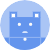search
Search
menu search toc more_vert
Guest 0reps
Thanks for the thanks!
close
account_circle
Profile
exit_to_app
Sign out
help Ask a question
search
keyboard_voice
close
Searching Tips
Search for a recipe:
"Creating a table in MySQL"
Search for an API documentation: "@append"
Search for code: "!dataframe"
Apply a tag filter: "#python"
Useful Shortcuts
/ to open search panel
Esc to close search panel
to navigate between search results
d to clear all current filters
Enter to expand content previewDoc SearchCode Search BetaSORRY NOTHING FOUND!
mic
Start speaking...Voice search is only supported in Safari and Chrome.
Shrink
Navigate to
A
A
brightness_medium
share
arrow_backShareTwitterFacebook

# NumPy | sum method

NumPy
chevron_right
Documentation
schedule Jul 1, 2022
Last updated
local_offer PythonNumPy
Tags

Numpy's `sum(~)` computes the sum of the values in the input array.

# Parameters

1. `a` | `array-like`

The input array for which you would like to compute the sum.

2. `axis`link | `None` or `int` | `optional`

The axis along which to compute the sum. For 2D arrays, the allowed values are as follows:

Axis

Meaning

0

Compute the sum column-wise

1

Compute the sum row-wise

None

Compute the sum of all values

By default, `axis=None`.

3. `dtype` | `string` or `type` | `optional`

The desired data type of the returned array. `dtype` will also be the type used during the computation of the sum. By default, the `dtype` of `a` is used.

4. `out`link | `Numpy array` | `optional`

Instead of creating a new array, you can place the computed sum into the array specified by `out`.

5. `initial`link | `scalar` | `optional`

The initial value used for the computation of the sum. By default, `initial=1`.

6. `where`link | `array` of `boolean` | `optional`

A boolean mask, where values that are flagged as False will be ignored, while those flagged as True will be used in the computation.

# Examples

## Basic usage

``` np.sum([1,2,3,4]) 10 ```

## Computing the sum of a 2D array

Consider the following 2D array:

``` a = np.array([[1,2],[3,4]])a array([[1, 2],       [3, 4]]) ```

### All values

``` np.sum(a) 10 ```

### Column-wise

``` np.sum(a, axis=0) array([4, 6]) ```

### Row-wise

``` np.sum(a, axis=1) array([3, 7]) ```

## Specifying an output array

``` a = np.zeros(2)np.sum([[1,2],[3,4]], axis=1, out=a)   # row-wise summationa array([3., 7.]) ```

Here, we've outputted the results to the array `a`.

## Specifying an initial value

``` np.sum([1,2,3], initial=10) 16 ```

Here, since we set an initial value of 10, we have `10+1+2+3=16`.

## Specifying a boolean mask

``` np.sum([4,5,6,7], where=[False, True, True, False]) 11 ```

Here, only the second and third values were included in the computation of the sum.

mail